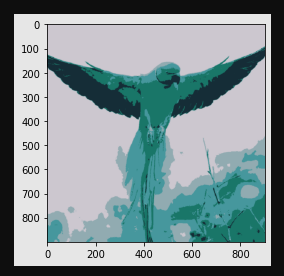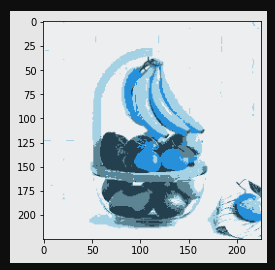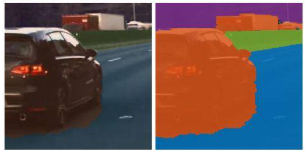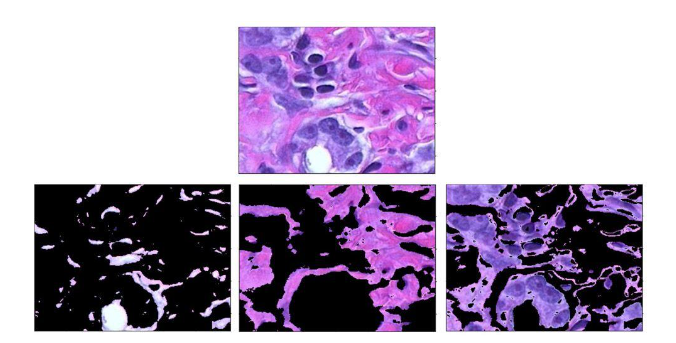# Image Segmentation by Clustering

## Introduction

Image Segmentation is the process of partitioning an image into multiple regions based on the characteristics of the pixels in the original image. Clustering is a technique to group similar entities and label them. Thus, for image segmentation using clustering, we can cluster similar pixels using a clustering algorithm and group a particular cluster pixel as a single segment.

Thus, let's explore more image segmentation using clustering,

### Image Segmentation

The process of image segmentation by clustering can be carried out using two methods.

• Agglomerative clustering

• Divisive clustering

In Agglomerative clustering, we label a pixel to a close cluster and then increase the size of the clusters iteratively. The following steps outline the process of Agglomerative clustering.

• Each pixel is considered to be an individual cluster

• Similar clusters with smaller inter-cluster distances (WCSS) are merged.

• The steps are repeated.

In Divisive clustering, the following process is followed.

• All the pixels are assigned to a single cluster.

• The cluster is split into two with large inter-cluster distance over some epochs.

• The steps are repeated until the optimal number of clusters is reached.

In this article, we are going to explore K-Means clustering for image segmentation.

## K-Means clustering for Image Segmentation

K-M eans is an agglomerative type of clustering where we don have the labels of pixels/data points beforehand. The number of clusters or groups formed is taken as K. These clusters are derived based on some of the similar or common characteristics between the pixels or data points.

The steps involved in K-Means clustering are

• Select a particular value of K

• A feature is taken in each pixel like RGB value, etc.

• Similar pixels are grouped by using distances like Euclidian distance.

• K-Means is used with the center of the cluster.

• Generally, a threshold is defined within which if the calculated size falls it is grouped into a single cluster.

## How to determine the value of K?

The objective function of the K-Means algorithm is WCSS.

$$\mathrm{C=\sum ^{M}_{i=1}} \:\:\mathrm{\sum ^{N}_{k=1}}\:\:\mathrm{|x^{i}_{k}-C_{i}|^{2}}$$

A graph is plotted between WCSS and K popularly known as the Elbow method. The point where the graph makes a sharp bend is noted and the corresponding value of K is taken. Determination of K is done using the Elbow method, a rather brute-force approach.

## Python Implementation

### Example

# KMEANS IMAGE SEGMENATION
import numpy as np
import cv2
import matplotlib.pyplot as plt
from sklearn.cluster import KMeans
%matplotlib inline

vector_1 = image_1.reshape((-1,3))
vector_2 = image_2.reshape((-1,3))
kmeans_1 = KMeans(n_clusters=5, random_state = 0, n_init=5).fit(vector_1)
c = np.uint8(kmeans_1.cluster_centers_)
seg_data= c[kmeans_1.labels_.flatten()]
seg_image = seg_data.reshape((image_1.shape))
plt.imshow(seg_image)
plt.pause(1)

kmeans_2 = KMeans(n_clusters=5, random_state = 0, n_init=5).fit(vector_2)
c = np.uint8(kmeans_2.cluster_centers_)
seg_data= c[kmeans_2.labels_.flatten()]
seg_image = seg_data.reshape((image_2.shape))
plt.imshow(seg_image)
plt.pause(1)


### Output## Application of Image Segmentation using K-Means clustering

• Autonomous vehicles − During the design of the perception system of self-driving vehicles semantic segmentation is used to separate objects from the surrounding in the vicinity of the car.• Imaging in Medical Field − Clustering is used to separate and identify types of tissues in cancerous cell images.• 3-D Scene Segmentation − In Robotic vision technology, systems are required to identify surrounding and localize objects. The Scene generated is 3D in nature. Clustering Algorithms are used for this purpose.

## Conclusion

Clustering is a very popular and effective technique for image segmentation. It can save valuable time while producing astonishing results for the majority of the use cases. In this regard, K-Means clustering stands as the winner.